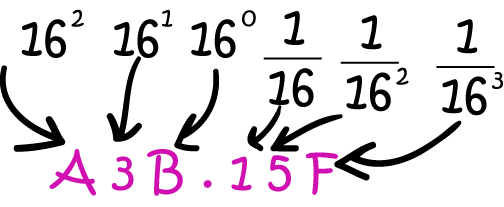# Math Definitions - Letter H

### ChaptersThe hexadecimal system is a number system based on the $16$ digits $\{0,1,2,3,4,5,6,7,8,9,A,B,C,D,E,F\}$.

Each digit of a hexadecimal number corresponds to a power of $16$, so it is a base-16 number system.

Computer programmers often use hexadecimal numbers to describe locations in the computer's memory.

### Description

The aim of this dictionary is to provide definitions to common mathematical terms. Students learn a new math skill every week at school, sometimes just before they start a new skill, if they want to look at what a specific term means, this is where this dictionary will become handy and a go-to guide for a student.

### Audience

Year 1 to Year 12 students

### Learning Objectives

Learn common math terms starting with letter H

Author: Subject Coach
You must be logged in as Student to ask a Question.